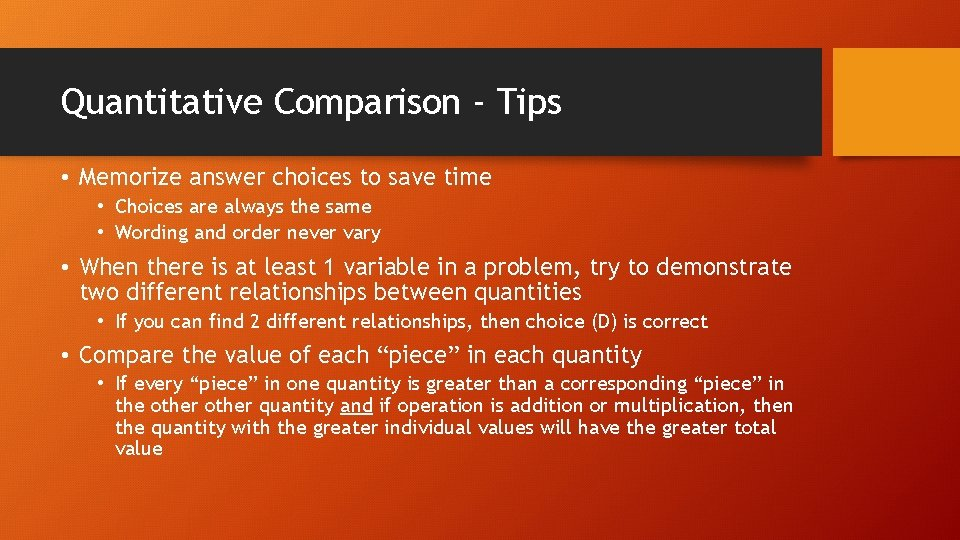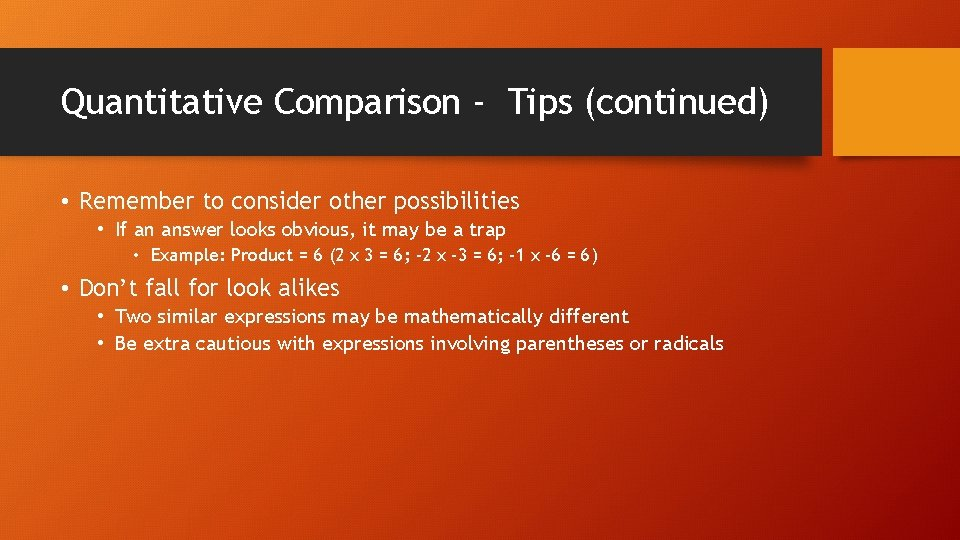# GRE QUANTITATIVE COMPARISON Quantitative Comparison Strategy Analyze Centered

• Slides: 5GRE QUANTITATIVE COMPARISONQuantitative Comparison - Strategy • Analyze Centered Information, Columns, and Quantities • Approach Strategically – variety of approaches to solving these questions [Note: Quantitative Comparison questions ask you to compare 2 mathematical expressions: Quantity A and Quantify B]Quantitative Comparison - Tips • Memorize answer choices to save time • Choices are always the same • Wording and order never vary • When there is at least 1 variable in a problem, try to demonstrate two different relationships between quantities • If you can find 2 different relationships, then choice (D) is correct • Compare the value of each “piece” in each quantity • If every “piece” in one quantity is greater than a corresponding “piece” in the other quantity and if operation is addition or multiplication, then the quantity with the greater individual values will have the greater total valueQuantitative Comparison – Tips (continued) • Make one quantity look like another • When quantities A and B are expressed differently, change the format of one quantity to match the other – this makes comparison easier • Do the same thing (operation) to both quantities • Simplify complex comparisons by performing addition, subtraction, multiplication, and division operations on both quantities to make them simpler • Don’t be tricked my misleading information • Don’t assume anything. Use only information that is given or information based on properties or theorems • For example: Don’t assume angles are equal or that all variables are positive integers – fractions or negative numbers impact relationship between quantitiesQuantitative Comparison - Tips (continued) • Remember to consider other possibilities • If an answer looks obvious, it may be a trap • Example: Product = 6 (2 x 3 = 6; -2 x -3 = 6; -1 x -6 = 6) • Don’t fall for look alikes • Two similar expressions may be mathematically different • Be extra cautious with expressions involving parentheses or radicals